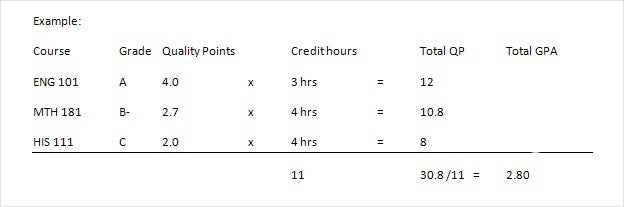# GPA example

Calculating your GPA requires two pieces of information:

1) the grade in each course and
2) the credit hours for each course.

The grades should then be converter to quality points. (e.g. A= 4.0, A- = 3.7, B+ = 3.3, etc).

Multiply the quality points times the credit hours

Total the quality points, Total the credit hours.

Divide the total quality points by the total credit hours.